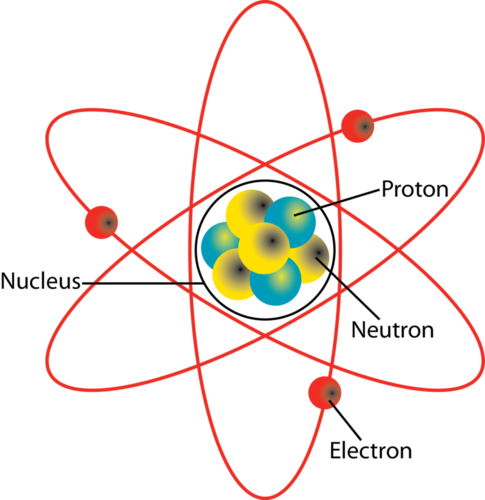Yourclasses: Best Preparation website for SSC, Banking & Railway Exams

# Chemistry : ATOMIC  STRUCTURE

Atom:-Atom is the smallest unit of matter which cannot be further divided.Atomic theory of matter:-Given by ‘John Dalton”

According to this theory:-

1.All matters are made up of atoms. Atoms are indivisible and indestructible.

1. All atoms of a given element are identical in mass and properties.

*Molecule: – It is the smallest particle of an element or compound that has a stable & independent existence.

Constituents of Atom: –

1. Electron – Discovered by J. J. Thomson

-Obtained from Cathode ray experiments.

1. Proton – Discovered by Goldstein & named by Rutherford.

– Obtained from Anode Ray Experiments.

1. Neutron – Discovered by James Chadwick.

– Charge on neutron is zero.

1. Isotopes -Elements having same atomic number but different mass number.
2. Isobars – Elements having same mass number but different atomic numbers.
3. Isotones – Atoms of different elements having same number of neutrons.

Note –Except Hydrogen atom, all atoms are madeup of 3 fundamental particles Ex.: Electron, Proton and Neutrons. Protons and Neutrons are present inside the Nucleus therefore are called Nucleons while electrons are present outside the nucleus.

Previous Year Questions based on above Topic:-

Q.1       The size of the nucleus is measured in :-

(a) Atomic mass unit

(b) Angstrom

(c) Cm

(d) Fermi

Exp. The size of the nucleus is measured in Fermi.

– Radius of atomic nucleus is of the order of

Q.2.Rutherford’s  alpha- scattering experiment related to the size of : –

(a) Nucleus

(b) Electron

(c) Atom

(d) Neutron

Ans. (a) Exp. – In this experiment, Rutherford bombarded the alpha- particle on a very thin gold foil. This leads to the discovery of the nucleus.

– Acc. To Rutherford’s atomic model, the electron inside an atom is No-stationary.

Q.3       The concept of dual nature of radiation was proposed by –

(a) Max plank

(b) De- Broglie.

(c) Pauling

(d) Heisenberg.

Ans. (b). Exp. –De-Broglie predicted that small particles such as electrons show wave like properties along with particle like character.

Note. – Heisenberg uncertainty principle : – Account to this principle- “The position and velocity of a small particle like electron cannot be simultaneously determined.

Q.4       The reverse effect of X-ray emission is : –

(a) Raman Effect

(b) Compton Effect

(c) Photo-electric effect

(d)Zeeman effect

Ans. (c) Exp. – In photoelectric effect, photons are incident on metal surface and electrons are produced whereas x-rays are produced when target metal is bombarded by electrons.

Note. :  X-Rays was discovered by Wilhelm Roentgen.

Q.5       Which of the following has lowest frequency?

(a) Visible light.

(b) X-Rays

(c) Gamma Rays

(d) Ultra violet Rays.

Ans. (A). Exp. –Types of radiations in decreasing order of frequencies or increasing order of wavelength are : –

Cosmic rays, Gamma Rays, Microwaves and Radio Waves.

Wavelength is inversely proportional to frequency.

Q.6       Total energy of revolving electron in an atom : –

(a) Cannot be negative

(b) will always  be positive

(c) can have any value above zero

(d) can never be positive.

Ans. (d) Total energy = Kinetic Energy + Potential energy.

m                     =         mass of electron

v                      =          velocity

r                       =          distance between nucleus and electron

K                     =          coulomb constant

e                      =        charge of electron

Z                     =          constant

Therefore, total energy of e can never be positive.

Q.7       Atomic mass no. is calculated using: –

(a) By total number of electrons.

(b) By adding number of protons and neutrons.

(c) By total number of protons.

(d) By total number of neutrons.

Ans. (b).

Q.8       Which of the following is an negatively charged ion?

(a) Calcium Ion

(b) Silver Ion

(c) Iodine Ion

(d) Zinc Ion

Ans. (c). Iodine ion is negatively charged as it is a nonmetal and gains electron to form anion whereas, Ca, Zn are metals, therefore they lose  e–  to form cations.

Q.9       Which of the following is not a cation?

(a) sulphate ion

(b) aluminum ion

(c) copper ion

(d) zinc ion

Exp. – Since, Aluminum, Copper & Zinc ions are metals, i.e. electron donor. Therefore, they loose electrons to form cation.

So, Sulphate ion is anion.

Q.10     Anions are formed by:-

(a) Losing of electrons.

(b) Gaining of neutrons

(c) Loosing of neutrons.

(d) Gaining of electrons.

Ans. (d). Exp. – Generally, Non-metals form anions to become stable by gaining of electrons.

#### You may also like...

This site uses Akismet to reduce spam. Learn how your comment data is processed.